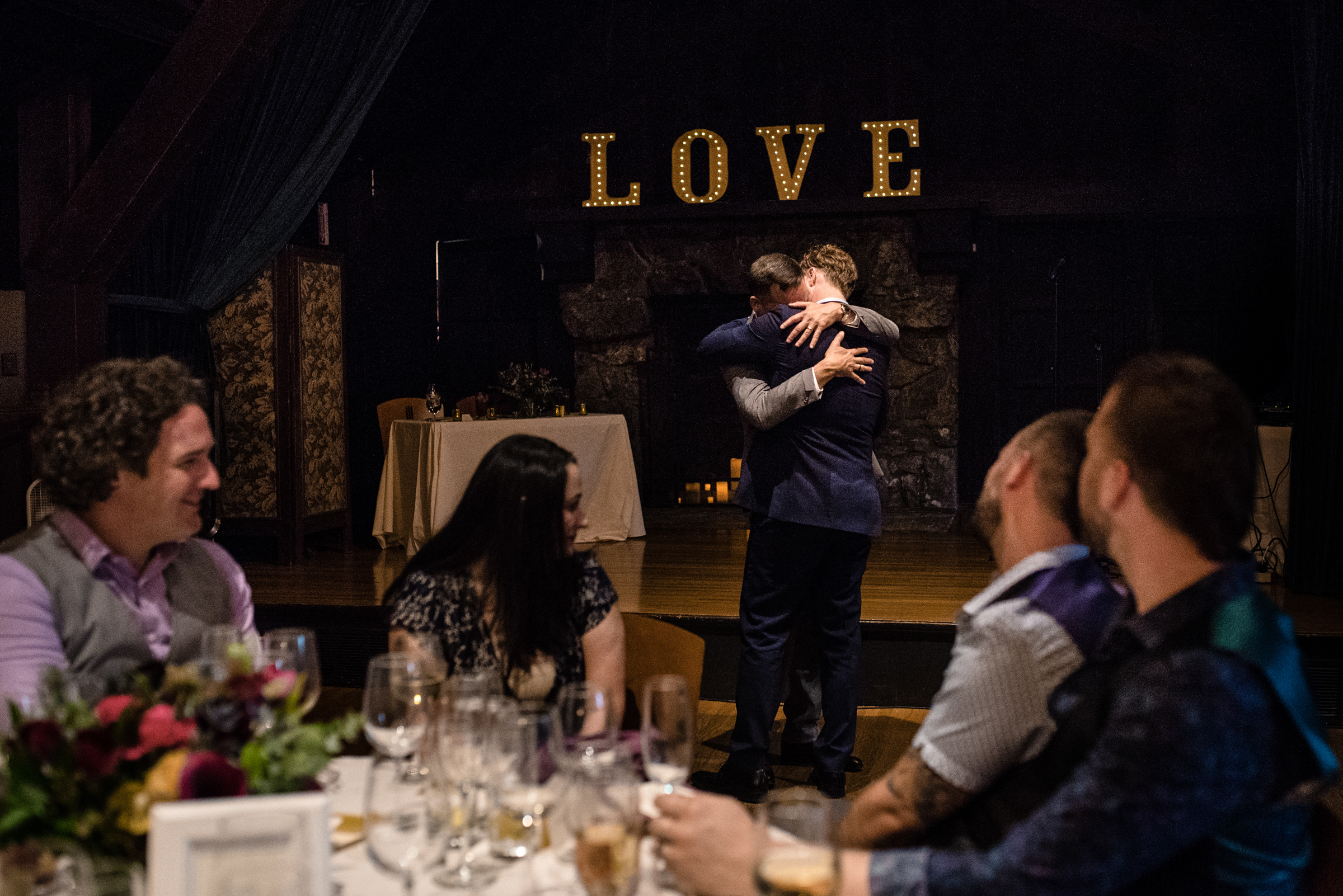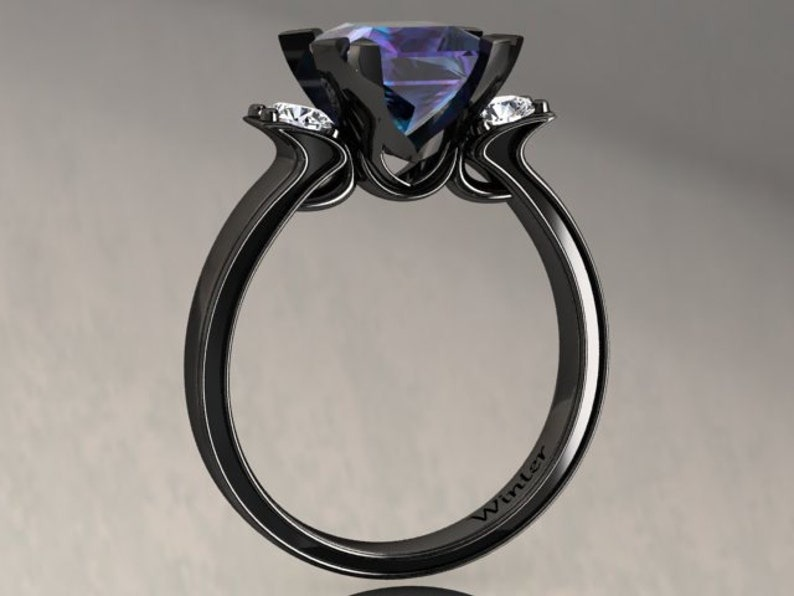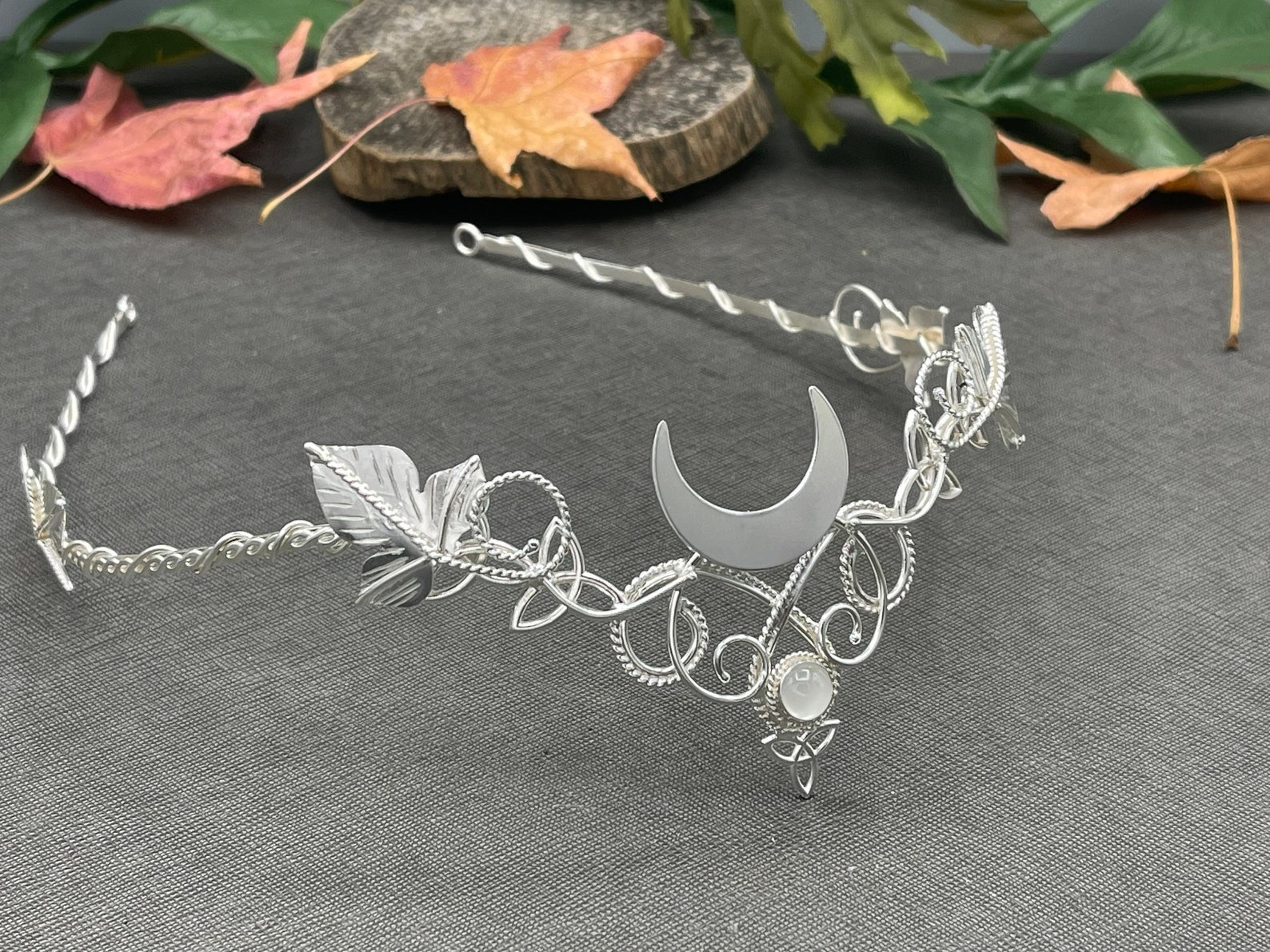# Christine & Matthew’s Good Vibes Anything Goes WeddingPosted by
``` Meet our fave wedding vendorsLinda Abbott PhotographyLos Angeles | PhotographyLisa Stone PhotographySan Francisco Bay Area | PhotographyTeton Wood BloomsOnline Vendors, Wyoming | Flowers & BouquetsAri Marie PhotographyConnecticut, Massachusetts, New Hampshire, Rhode Island | Photography New in our curated shopFunny Marriage Congratulations CardTrending Gifts & Favors | Etsy, TrapCardStudioAlexandrite 2.00 Carat Princess Cut Black Gold RingTrending Engagement Rings | Etsy, WinterFineJewelryCrown with antlersTrending Accessories | Etsy, MagaelaAccessoriesCeltic Knot Moon TiaraTrending Accessories | Camias, Etsy Comments on Christine & Matthew’s Good Vibes Anything Goes WeddingKristen says: Feb. 3 2009 at 11:52 am I freaking love that Beatles cake!Jen says: Feb. 3 2009 at 12:27 pm One of the best weddings I have been to (next to my own of course!). Nothing like attending a wedding where you know down to every detail, it’s the perfect representation of who they are and how much they love each other!!Christine says: Feb. 3 2009 at 12:29 pm Wow! I just about screamed when I saw our wedding on here! What an honor to be on the OBB blog! Thanks!!!Erica says: Feb. 3 2009 at 12:30 pm I love the Beatles cake!! Yellow Submarine was the first full song that I ever sang and I am walking down the aisle to “All you need is love”.Aurora says: Feb. 3 2009 at 1:08 pm Oh my gosh, I love her hair! Very Audrey indeed!Katie says: Feb. 3 2009 at 2:51 pm this is probably one of the most inspirational weddings i’ve seen. it’s cute and romantic in its own little way, while still staying true to the couple. and it looks like it was tons of fun to be there. definitely what i’m going for for my own wedding.Pam says: Feb. 3 2009 at 4:22 pm WoW! How exciting is this! The wedding was fun but this is terrific!Alex says: Feb. 3 2009 at 4:43 pm Where did those great shoes come from? Love them!Missy says: Feb. 3 2009 at 5:11 pm It’s great going back to that special day. I was glad to be a part of it.Lauren says: Feb. 3 2009 at 5:27 pm I freakin’ LOVE this wedding!!! Every detail of it is so unique and personal. Love it…Love it…LOVE IT!!!kim says: Feb. 3 2009 at 5:51 pm Darling darling darling!!! That Beatles cake is the bomb!!!Bonnie says: Feb. 3 2009 at 9:25 pm AWESOME bouquet!!! 🙂Carole Zacek says: Feb. 6 2009 at 7:28 am OH CHRISTINE!!! Thank YOU for letting me be part of this!! It was great to work with such a creative gal on your dress!!! THANKS AGAIN!! CaroleLiza says: Feb. 7 2009 at 12:48 am That cake is adorable! (Has anyone ever told your husband that he looks like Doctor Who? It’s uncanny!)Kendra says: Feb. 23 2009 at 10:50 am Okay, seriously, I need to get in touch with you. Im getting married on June 6th, 2009. I live in Bellevue, NE! (wow) And guess what, we’re having a Beatles themed wedding. CRAZY! I just happened to be searching the internet looking to see if anyone had anything remotely like a Beatles wedding. Well I found you, and you probably live like, five minutes away. Thats just too cool.Christine says: Mar. 1 2009 at 6:15 am Kendra!!! Send me a message on the OBT! I want to hear all about your wedding.arrielle says: Mar. 26 2009 at 4:43 pm This is the cutest most goregous wedding ever. i wasnt there but i can see the love and joy and all other emotions u two have for each other it made me cry. =]Darcy says: Apr. 18 2009 at 2:29 am Hello i think your wedding from what i can tell was awesome! I myself want a retro wedding and im having trouble trying to figure out everything! If you have any ideas. Thank you and i love your Wifes Hair it so adorable.Alison says: Jul. 29 2009 at 6:50 pm I loveee the cake! amazing. i deff want a cake like that for my weddingDy says: Mar. 15 2010 at 6:06 pm BEAUTIFUL!!!!! Comments are closed. ```
``` ```
```About Us Offbeat Wed celebrates folks daring to walk off the beaten aisle. We're here to amplify the visibility of those who feel left out of traditional wedding media. Published by Offbeat Bride author Ariel Meadow Stallings, now with Suki Lanh and Yelahneb. SponsorsFor our vendor friends:Reach more offbeat clients! ```
``` ```
``` SubscribeOur newsletter is the best way to keep up with us — we’ll email you weekly with tools, advice, inspo, discounts, and more! Email NameThis field is for validation purposes and should be left unchanged. Δ Going viral todayOfficiating a wedding?LEARN MORE About Us Offbeat Wed launched in January 2007, supporting the release of Ariel Meadow Stallings' book, Offbeat Bride. We now have over 7k posts and have helped 60 million nontraditional folks plan weddings full of intention and personality. LEARN MORE GET IN TOUCHNew Reader? Advertise Submissions Contact Archives About Us Copyright © 2007 - 2023 Offbeat Empire. All rights reserved. Unauthorized reproduction in part or in whole is prohibited. Terms of Service Privacy Policy Monetized by SkimLinks ```
``` adthrive.cmd.push(function () { // Disable all ads if the URL matches any of the patterns (Uses Regular Expressions) adthrive.disableAds(['/shop', '/item', '/?shop', '/seller', '/vendor-category']); }); 'use strict';(function(){function x(c,a){function b(){this.constructor=c}if("function"!==typeof a&&null!==a)throw new TypeError("Class extends value "+String(a)+" is not a constructor or null");S(c,a);c.prototype=null===a?Object.create(a):(b.prototype=a.prototype,new b)}function Y(c,a){var b={},d;for(d in c)Object.prototype.hasOwnProperty.call(c,d)&&0>a.indexOf(d)&&(b[d]=c[d]);if(null!=c&&"function"===typeof Object.getOwnPropertySymbols){var e=0;for(d=Object.getOwnPropertySymbols(c);e<d.length;e++)0> a.indexOf(d[e])&&Object.prototype.propertyIsEnumerable.call(c,d[e])&&(b[d[e]]=c[d[e]])}return b}function J(c,a,b,d){var e=arguments.length,f=3>e?a:null===d?d=Object.getOwnPropertyDescriptor(a,b):d,g;if("object"===typeof Reflect&&"function"===typeof Reflect.decorate)f=Reflect.decorate(c,a,b,d);else for(var h=c.length-1;0<=h;h--)if(g=c[h])f=(3>e?g(f):3<e?g(a,b,f):g(a,b))||f;return 3<e&&f&&Object.defineProperty(a,b,f),f}function E(c,a){if("object"===typeof Reflect&&"function"===typeof Reflect.metadata)return Reflect.metadata(c, a)}function Q(c){var a="function"===typeof Symbol&&Symbol.iterator,b=a&&c[a],d=0;if(b)return b.call(c);if(c&&"number"===typeof c.length)return{next:function(){c&&d>=c.length&&(c=void 0);return{value:c&&c[d++],done:!c}}};throw new TypeError(a?"Object is not iterable.":"Symbol.iterator is not defined.");}function u(c,a){var b="function"===typeof Symbol&&c[Symbol.iterator];if(!b)return c;c=b.call(c);var d,e=[];try{for(;(void 0===a||0<a--)&&!(d=c.next()).done;)e.push(d.value)}catch(g){var f={error:g}}finally{try{d&& !d.done&&(b=c["return"])&&b.call(c)}finally{if(f)throw f.error;}}return e}function C(c,a,b){if(b||2===arguments.length)for(var d=0,e=a.length,f;d<e;d++)!f&&d in a||(f||(f=Array.prototype.slice.call(a,0,d)),f[d]=a[d]);return c.concat(f||Array.prototype.slice.call(a))}function T(c,a){void 0===a&&(a={});a=a.insertAt;if(c&&"undefined"!==typeof document){var b=document.head||document.getElementsByTagName("head"),d=document.createElement("style");d.type="text/css";"top"===a?b.firstChild?b.insertBefore(d, b.firstChild):b.appendChild(d):b.appendChild(d);d.styleSheet?d.styleSheet.cssText=c:d.appendChild(document.createTextNode(c))}}window.adthriveCLS.buildDate="2023-11-29";var S=function(c,a){S=Object.setPrototypeOf||{__proto__:[]}instanceof Array&&function(b,d){b.__proto__=d}||function(b,d){for(var e in d)Object.prototype.hasOwnProperty.call(d,e)&&(b[e]=d[e])};return S(c,a)},z=function(){z=Object.assign||function(c){for(var a,b=1,d=arguments.length;b<d;b++){a=arguments[b];for(var e in a)Object.prototype.hasOwnProperty.call(a, e)&&(c[e]=a[e])}return c};return z.apply(this,arguments)},q=new (function(){function c(){}c.prototype.info=function(a,b){for(var d=[],e=2;e<arguments.length;e++)d[e-2]=arguments[e];this.call.apply(this,C([console.info,a,b],u(d),!1))};c.prototype.warn=function(a,b){for(var d=[],e=2;e<arguments.length;e++)d[e-2]=arguments[e];this.call.apply(this,C([console.warn,a,b],u(d),!1))};c.prototype.error=function(a,b){for(var d=[],e=2;e<arguments.length;e++)d[e-2]=arguments[e];this.call.apply(this,C([console.error, a,b],u(d),!1));this.sendErrorLogToCommandQueue.apply(this,C([a,b],u(d),!1))};c.prototype.event=function(a,b){for(var d,e=2;e<arguments.length;e++);"debug"===(null===(d=window.adthriveCLS)||void 0===d?void 0:d.bucket)&&this.info(a,b)};c.prototype.sendErrorLogToCommandQueue=function(a,b){for(var d=[],e=2;e<arguments.length;e++)d[e-2]=arguments[e];window.adthrive=window.adthrive||{};window.adthrive.cmd=window.adthrive.cmd||[];window.adthrive.cmd.push(function(){void 0!==window.adthrive.logError&&"function"=== typeof window.adthrive.logError&&window.adthrive.logError(a,b,d)}.bind(a,b,d))};c.prototype.call=function(a,b,d){for(var e=[],f=3;f<arguments.length;f++)e[f-3]=arguments[f];f=["%c".concat(b,"::").concat(d," ")];var g=["color: #999; font-weight: bold;"];0<e.length&&"string"===typeof e&&f.push(e.shift());g.push.apply(g,C([],u(e),!1));try{Function.prototype.apply.call(a,console,C([f.join("")],u(g),!1))}catch(h){console.error(h)}};return c}()),w=function(c,a){return null==c||c!==c?a:c},pa=function(c){var a= c.clientWidth;getComputedStyle&&(c=getComputedStyle(c,null),a-=parseFloat(c.paddingLeft||"0")+parseFloat(c.paddingRight||"0"));return a},Z=function(c){var a=c.offsetHeight,b=c.offsetWidth,d=c.getBoundingClientRect(),e=document.body,f=document.documentElement;c=Math.round(d.top+(window.pageYOffset||f.scrollTop||e.scrollTop)-(f.clientTop||e.clientTop||0));d=Math.round(d.left+(window.pageXOffset||f.scrollLeft||e.scrollLeft)-(f.clientLeft||e.clientLeft||0));return{top:c,left:d,bottom:c+a,right:d+b,width:b, height:a}},G=function(){var c=navigator.userAgent,a=/Tablet|iPad|Playbook|Nook|webOS|Kindle|Android (?!.*Mobile).*Safari|CrOS/i.test(c);return/Mobi|iP(hone|od)|Opera Mini/i.test(c)&&!a},qa=function(c){void 0===c&&(c=document);return(c===document?document.body:c).getBoundingClientRect().top},ra=function(c){return c.includes(",")?c.split(","):[c]},sa=function(c){void 0===c&&(c=document);c=c.querySelectorAll("article");return 0===c.length?null:(c=Array.from(c).reduce(function(a,b){return b.offsetHeight> a.offsetHeight?b:a}))&&c.offsetHeight>1.5*window.innerHeight?c:null},ta=function(c,a,b){void 0===b&&(b=document);var d=sa(b),e=d?[d]:[],f=[];c.forEach(function(h){var k=Array.from(b.querySelectorAll(h.elementSelector)).slice(0,h.skip);ra(h.elementSelector).forEach(function(l){var n=b.querySelectorAll(l);l=function(r){var m=n[r];if(a.map.some(function(t){return t.el.isEqualNode(m)}))return"continue";(r=m&&m.parentElement)&&r!==document.body?e.push(r):e.push(m);-1===k.indexOf(m)&&f.push({dynamicAd:h, element:m})};for(var p=0;p<n.length;p++)l(p)})});var g=qa(b);c=f.sort(function(h,k){return h.element.getBoundingClientRect().top-g-(k.element.getBoundingClientRect().top-g)});return[e,c]},ua=function(c,a,b){void 0===b&&(b=document);a=u(ta(c,a,b),2);c=a;a=a;if(0===c.length)throw Error("No Main Content Elements Found");return[Array.from(c).reduce(function(d,e){return e.offsetHeight>d.offsetHeight?e:d})||document.body,a]},A;(function(c){c.amznbid="amznbid";c.amzniid="amzniid";c.amznp="amznp";c.amznsz= "amznsz"})(A||(A={}));var H;(function(c){c.ThirtyThreeAcross="33across";c.AppNexus="appnexus";c.Amazon="amazon";c.Colossus="colossus";c.ColossusServer="col_ss";c.Conversant="conversant";c.Concert="concert";c.Criteo="criteo";c.GumGum="gumgum";c.ImproveDigital="improvedigital";c.ImproveDigitalServer="improve_ss";c.IndexExchange="ix";c.Kargo="kargo";c.KargoServer="krgo_ss";c.MediaGrid="grid";c.MediaGridVideo="gridvid";c.Nativo="nativo";c.OpenX="openx";c.Ogury="ogury";c.OpenXServer="opnx_ss";c.Pubmatic= "pubmatic";c.PubmaticServer="pubm_ss";c.ResetDigital="resetdigital";c.Roundel="roundel";c.Rtbhouse="rtbhouse";c.Rubicon="rubicon";c.RubiconServer="rubi_ss";c.Sharethrough="sharethrough";c.Teads="teads";c.Triplelift="triplelift";c.TripleliftServer="tripl_ss";c.TTD="ttd";c.Undertone="undertone";c.UndertoneServer="under_ss";c.Unruly="unruly";c.YahooSSP="yahoossp";c.YahooSSPServer="yah_ss";c.Verizon="verizon";c.Yieldmo="yieldmo"})(H||(H={}));var aa;(function(c){c.Prebid="prebid";c.GAM="gam";c.Amazon= "amazon";c.Marmalade="marmalade";c.Floors="floors";c.CMP="cmp"})(aa||(aa={}));var ba;(function(c){c.cm="cm";c.fbrap="fbrap";c.rapml="rapml"})(ba||(ba={}));var ca;(function(c){c.lazy="lazy";c.raptive="raptive";c.refresh="refresh";c.session="session";c.crossDomain="crossdomain";c.highSequence="highsequence";c.lazyBidPool="lazyBidPool"})(ca||(ca={}));var da;(function(c){c.lazy="l";c.raptive="rapml";c.refresh="r";c.session="s";c.crossdomain="c";c.highsequence="hs";c.lazyBidPool="lbp"})(da||(da={}));var ea; (function(c){c.Version="Version";c.SharingNotice="SharingNotice";c.SaleOptOutNotice="SaleOptOutNotice";c.SharingOptOutNotice="SharingOptOutNotice";c.TargetedAdvertisingOptOutNotice="TargetedAdvertisingOptOutNotice";c.SensitiveDataProcessingOptOutNotice="SensitiveDataProcessingOptOutNotice";c.SensitiveDataLimitUseNotice="SensitiveDataLimitUseNotice";c.SaleOptOut="SaleOptOut";c.SharingOptOut="SharingOptOut";c.TargetedAdvertisingOptOut="TargetedAdvertisingOptOut";c.SensitiveDataProcessing="SensitiveDataProcessing"; c.KnownChildSensitiveDataConsents="KnownChildSensitiveDataConsents";c.PersonalDataConsents="PersonalDataConsents";c.MspaCoveredTransaction="MspaCoveredTransaction";c.MspaOptOutOptionMode="MspaOptOutOptionMode";c.MspaServiceProviderMode="MspaServiceProviderMode";c.SubSectionType="SubsectionType";c.Gpc="Gpc"})(ea||(ea={}));var fa;(function(c){c[c.NA=0]="NA";c[c.OptedOut=1]="OptedOut";c[c.OptedIn=2]="OptedIn"})(fa||(fa={}));var F;(function(c){c.AdDensity="addensity";c.AdLayout="adlayout";c.FooterCloseButton= "footerclose";c.Interstitial="interstitial";c.RemoveVideoTitleWrapper="removevideotitlewrapper";c.StickyOutstream="stickyoutstream";c.StickyOutstreamOnStickyPlayer="sospp";c.VideoAdvancePlaylistRelatedPlayer="videoadvanceplaylistrp";c.MobileStickyPlayerPosition="mspp"})(F||(F={}));var M;(function(c){c.Desktop="desktop";c.Mobile="mobile"})(M||(M={}));var L;(function(c){c.Video_Collapse_Autoplay_SoundOff="Video_Collapse_Autoplay_SoundOff";c.Video_Individual_Autoplay_SOff="Video_Individual_Autoplay_SOff"; c.Video_Coll_SOff_Smartphone="Video_Coll_SOff_Smartphone";c.Video_In_Post_ClicktoPlay_SoundOn="Video_In-Post_ClicktoPlay_SoundOn"})(L||(L={}));var ha;(ha||(ha={})).None="none";var va=function(c,a){var b=c.adDensityEnabled;c=c.adDensityLayout.pageOverrides.find(function(d){return!!document.querySelector(d.pageSelector)&&(d[a].onePerViewport||"number"===typeof d[a].adDensity)});return b?!c:!0};A=function(){function c(){this._timeOrigin=0}c.prototype.resetTimeOrigin=function(){this._timeOrigin=window.performance.now()}; c.prototype.now=function(){try{return Math.round(window.performance.now()-this._timeOrigin)}catch(a){return 0}};return c}();window.adthrive.windowPerformance=window.adthrive.windowPerformance||new A;A=window.adthrive.windowPerformance;var U=A.now.bind(A),wa=function(c){void 0===c&&(c=window.location.search);var a=0===c.indexOf("?")?1:0;return c.slice(a).split("&").reduce(function(b,d){d=u(d.split("="),2);b.set(d,d);return b},new Map)},ia=function(c){try{return{valid:!0,elements:document.querySelectorAll(c)}}catch(a){return z({valid:!1}, a)}},V=function(c){return""===c?{valid:!0}:ia(c)},xa=function(c){var a=c.reduce(function(b,d){return d.weight?d.weight+b:b},0);return 0<c.length&&c.every(function(b){var d=b.value;b=b.weight;return!(void 0===d||null===d||"number"===typeof d&&isNaN(d)||!b)})&&100===a},ya=["siteId","siteName","adOptions","breakpoints","adUnits"],za=function(c){var a={},b=wa().get(c);if(b)try{var d=decodeURIComponent(b);a=JSON.parse(d);q.event("ExperimentOverridesUtil","getExperimentOverrides",c,a)}catch(e){}return a}, Aa=function(c){function a(b){var d=c.call(this)||this;d._featureRollouts=b.enabled?b.siteAds.featureRollouts||{}:{};return d}x(a,c);return a}(function(){function c(){this._featureRollouts={}}Object.defineProperty(c.prototype,"siteFeatureRollouts",{get:function(){return this._featureRollouts},enumerable:!1,configurable:!0});c.prototype.isRolloutEnabled=function(a){return this._featureRollouts&&this._featureRollouts[a]?this._featureRollouts[a].enabled:!1};return c}()),ja=function(){function c(){this._clsGlobalData= window.adthriveCLS}Object.defineProperty(c.prototype,"enabled",{get:function(){var a;if(a=!!this._clsGlobalData&&!!this._clsGlobalData.siteAds)a:{a=this._clsGlobalData.siteAds;var b=void 0;void 0===b&&(b=ya);if(a){for(var d=0;d<b.length;d++)if(!a[b[d]]){a=!1;break a}a=!0}else a=!1}return a},enumerable:!1,configurable:!0});Object.defineProperty(c.prototype,"error",{get:function(){return!(!this._clsGlobalData||!this._clsGlobalData.error)},enumerable:!1,configurable:!0});Object.defineProperty(c.prototype, "siteAds",{get:function(){return this._clsGlobalData.siteAds},set:function(a){this._clsGlobalData.siteAds=a},enumerable:!1,configurable:!0});Object.defineProperty(c.prototype,"disableAds",{get:function(){return this._clsGlobalData.disableAds},set:function(a){this._clsGlobalData.disableAds=a},enumerable:!1,configurable:!0});Object.defineProperty(c.prototype,"enabledLocations",{get:function(){return this._clsGlobalData.enabledLocations},set:function(a){this._clsGlobalData.enabledLocations=a},enumerable:!1, configurable:!0});Object.defineProperty(c.prototype,"injectedFromPlugin",{get:function(){return this._clsGlobalData.injectedFromPlugin},set:function(a){this._clsGlobalData.injectedFromPlugin=a},enumerable:!1,configurable:!0});Object.defineProperty(c.prototype,"injectedFromSiteAds",{get:function(){return this._clsGlobalData.injectedFromSiteAds},set:function(a){this._clsGlobalData.injectedFromSiteAds=a},enumerable:!1,configurable:!0});c.prototype.overwriteInjectedSlots=function(a){this._clsGlobalData.injectedSlots= a};c.prototype.setInjectedSlots=function(a){this._clsGlobalData.injectedSlots=this._clsGlobalData.injectedSlots||[];this._clsGlobalData.injectedSlots.push(a)};Object.defineProperty(c.prototype,"injectedSlots",{get:function(){return this._clsGlobalData.injectedSlots},enumerable:!1,configurable:!0});c.prototype.setInjectedVideoSlots=function(a){this._clsGlobalData.injectedVideoSlots=this._clsGlobalData.injectedVideoSlots||[];this._clsGlobalData.injectedVideoSlots.push(a)};Object.defineProperty(c.prototype, "injectedVideoSlots",{get:function(){return this._clsGlobalData.injectedVideoSlots},enumerable:!1,configurable:!0});c.prototype.setInjectedScripts=function(a){this._clsGlobalData.injectedScripts=this._clsGlobalData.injectedScripts||[];this._clsGlobalData.injectedScripts.push(a)};Object.defineProperty(c.prototype,"getInjectedScripts",{get:function(){return this._clsGlobalData.injectedScripts},enumerable:!1,configurable:!0});c.prototype.setExperiment=function(a,b,d){void 0===d&&(d=!1);this._clsGlobalData.experiments= this._clsGlobalData.experiments||{};this._clsGlobalData.siteExperiments=this._clsGlobalData.siteExperiments||{};(d?this._clsGlobalData.siteExperiments:this._clsGlobalData.experiments)[a]=b};c.prototype.getExperiment=function(a,b){void 0===b&&(b=!1);return(b=b?this._clsGlobalData.siteExperiments:this._clsGlobalData.experiments)&&b[a]};c.prototype.setWeightedChoiceExperiment=function(a,b,d){void 0===d&&(d=!1);this._clsGlobalData.experimentsWeightedChoice=this._clsGlobalData.experimentsWeightedChoice|| {};this._clsGlobalData.siteExperimentsWeightedChoice=this._clsGlobalData.siteExperimentsWeightedChoice||{};(d?this._clsGlobalData.siteExperimentsWeightedChoice:this._clsGlobalData.experimentsWeightedChoice)[a]=b};c.prototype.getWeightedChoiceExperiment=function(a,b){var d,e;void 0===b&&(b=!1);return(b=b?null===(d=this._clsGlobalData)||void 0===d?void 0:d.siteExperimentsWeightedChoice:null===(e=this._clsGlobalData)||void 0===e?void 0:e.experimentsWeightedChoice)&&b[a]};Object.defineProperty(c.prototype, "branch",{get:function(){return this._clsGlobalData.branch},enumerable:!1,configurable:!0});Object.defineProperty(c.prototype,"bucket",{get:function(){return this._clsGlobalData.bucket},enumerable:!1,configurable:!0});Object.defineProperty(c.prototype,"videoDisabledFromPlugin",{get:function(){return this._clsGlobalData.videoDisabledFromPlugin},set:function(a){this._clsGlobalData.videoDisabledFromPlugin=a},enumerable:!1,configurable:!0});Object.defineProperty(c.prototype,"targetDensityLog",{get:function(){return this._clsGlobalData.targetDensityLog}, set:function(a){this._clsGlobalData.targetDensityLog=a},enumerable:!1,configurable:!0});c.prototype.shouldHalveIOSDensity=function(){var a=new Aa(this),b=void 0;void 0===b&&(b=navigator.userAgent);return/iP(hone|od|ad)/i.test(b)&&a.isRolloutEnabled("iOS-Resolution")};c.prototype.getTargetDensity=function(a){return this.shouldHalveIOSDensity()?a/2:a};Object.defineProperty(c.prototype,"removeVideoTitleWrapper",{get:function(){return this._clsGlobalData.siteAds.adOptions.removeVideoTitleWrapper},enumerable:!1, configurable:!0});return c}(),Ba=function(){function c(){}c.getScrollTop=function(){return(window.pageYOffset||document.documentElement.scrollTop)-(document.documentElement.clientTop||0)};c.getScrollBottom=function(){return this.getScrollTop()+(document.documentElement.clientHeight||0)};c.shufflePlaylist=function(a){for(var b=a.length,d,e;0!==b;)e=Math.floor(Math.random()*a.length),--b,d=a[b],a[b]=a[e],a[e]=d;return a};c.isMobileLandscape=function(){return window.matchMedia("(orientation: landscape) and (max-height: 480px)").matches}; c.playerViewable=function(a){a=a.getBoundingClientRect();return this.isMobileLandscape()?window.innerHeight>a.top+a.height/2&&0<a.top+a.height/2:window.innerHeight>a.top+a.height/2};c.createQueryString=function(a){return Object.keys(a).map(function(b){return"".concat(b,"=").concat(a[b])}).join("&")};c.createEncodedQueryString=function(a){return Object.keys(a).map(function(b){return"".concat(b,"=").concat(encodeURIComponent(a[b]))}).join("&")};c.setMobileLocation=function(a){a=a||"bottom-right";"top-left"=== a?a="adthrive-collapse-top-left":"top-right"===a?a="adthrive-collapse-top-right":"bottom-left"===a?a="adthrive-collapse-bottom-left":"bottom-right"===a?a="adthrive-collapse-bottom-right":"top-center"===a&&(a=G()?"adthrive-collapse-top-center":"adthrive-collapse-bottom-right");return a};c.addMaxResolutionQueryParam=function(a){var b=G()?"320":"1280";b="max_resolution=".concat(b);var d=u(String(a).split("?"),2);a=d;b=(d=d)?d+"&".concat(b):b;return"".concat(a,"?").concat(b)};return c}(),Ca=function(){return function(c){this._clsOptions= c;this.removeVideoTitleWrapper=w(this._clsOptions.siteAds.adOptions.removeVideoTitleWrapper,!1);c=this._clsOptions.siteAds.videoPlayers;this.footerSelector=w(c&&c.footerSelector,"");this.players=w(c&&c.players.map(function(a){a.mobileLocation=Ba.setMobileLocation(a.mobileLocation);return a}),[]);this.contextualSettings=c&&c.contextual}}(),Da=function(){return function(c){this.contextualPlayerAdded=this.playlistPlayerAdded=this.mobileStickyPlayerOnPage=!1;this.footerSelector="";this.removeVideoTitleWrapper= !1;this.videoAdOptions=new Ca(c);this.players=this.videoAdOptions.players;this.contextualSettings=this.videoAdOptions.contextualSettings;this.removeVideoTitleWrapper=this.videoAdOptions.removeVideoTitleWrapper;this.footerSelector=this.videoAdOptions.footerSelector}}();H=function(){return function(){}}();var I=function(c){function a(b){var d=c.call(this)||this;d._probability=b;return d}x(a,c);a.prototype.get=function(){if(0>this._probability||1<this._probability)throw Error("Invalid probability: ".concat(this._probability)); return Math.random()<this._probability};return a}(H);A=function(){function c(){this._clsOptions=new ja;this.shouldUseCoreExperimentsConfig=!1}c.prototype.setExperimentKey=function(a){void 0===a&&(a=!1);this._clsOptions.setExperiment(this.abgroup,this.result,a)};return c}();var Ea=function(c){function a(){var b=c.call(this)||this;b._result=!1;b._choices=[{choice:!0},{choice:!1}];b.key="RemoveLargeSize";b.abgroup="smhd100";b._result=b.run();b.setExperimentKey();return b}x(a,c);Object.defineProperty(a.prototype, "result",{get:function(){return this._result},enumerable:!1,configurable:!0});a.prototype.run=function(){return(new I(.1)).get()};return a}(A),ka=function(c,a,b,d,e,f){c=Math.round(f-e);a=[];e=[];a.push("(",b.map(function(){return"%o"}).join(", "),")");e.push.apply(e,C([],u(b),!1));void 0!==d&&(a.push(" => %o"),e.push(d));a.push(" %c(".concat(c,"ms)"));e.push("color: #999;")},la=function(c,a,b){var d=void 0!==b.get?b.get:b.value;return function(){for(var e=[],f=0;f<arguments.length;f++)e[f]=arguments[f]; try{var g=U(),h=d.apply(this,e);if(h instanceof Promise)return h.then(function(l){var n=U();ka(c,a,e,l,g,n);return Promise.resolve(l)}).catch(function(l){l.logged||(q.error(c,a,l),l.logged=!0);throw l;});var k=U();ka(c,a,e,h,g,k);return h}catch(l){throw l.logged||(q.error(c,a,l),l.logged=!0),l;}}},N=function(c,a){void 0===a&&(a=!1);return function(b){var d,e=Object.getOwnPropertyNames(b.prototype).filter(function(p){return a||0!==p.indexOf("_")}).map(function(p){return[p,Object.getOwnPropertyDescriptor(b.prototype, p)]});try{for(var f=Q(e),g=f.next();!g.done;g=f.next()){var h=u(g.value,2),k=h,l=h;void 0!==l&&"function"===typeof l.value?b.prototype[k]=la(c,k,l):void 0!==l&&void 0!==l.get&&"function"===typeof l.get&&Object.defineProperty(b.prototype,k,z(z({},l),{get:la(c,k,l)}))}}catch(p){var n={error:p}}finally{try{g&&!g.done&&(d=f.return)&&d.call(f)}finally{if(n)throw n.error;}}}},Fa=function(c){function a(){var b=c.call(this)||this;b._result=!1;b.key="MaxContent";b.abgroup="conmax99";b._choices=[{choice:!0}, {choice:!1}];b.weight=.02;b._result=b.run();b.setExperimentKey();return b}x(a,c);Object.defineProperty(a.prototype,"result",{get:function(){return this._result},enumerable:!1,configurable:!0});a.prototype.run=function(){return(new I(this.weight)).get()};return a=J([N("MaxContentExperiment"),E("design:paramtypes",[])],a)}(A),Ga=function(c){function a(b){var d=c.call(this)||this;d._result=!1;d.key="ParallaxAdsExperiment";d.abgroup="parallax";d._choices=[{choice:!0},{choice:!1}];d.weight=.5;G()&&b.largeFormatsMobile&& (d._result=d.run(),d.setExperimentKey());return d}x(a,c);Object.defineProperty(a.prototype,"result",{get:function(){return this._result},enumerable:!1,configurable:!0});a.prototype.run=function(){return(new I(this.weight)).get()};return a=J([N("ParallaxAdsExperiment"),E("design:paramtypes",[Object])],a)}(A),Ha=function(c){function a(){var b=c.call(this)||this;b._result=!1;b._choices=[{choice:!0},{choice:!1}];b.key="mrsf";b.abgroup="mrsf";G()&&(b._result=b.run(),b.setExperimentKey());return b}x(a, c);Object.defineProperty(a.prototype,"result",{get:function(){return this._result},enumerable:!1,configurable:!0});a.prototype.run=function(){return(new I(1)).get()};return a}(A),Ia=[[728,90],[300,250],[300,600],[320,50],[970,250],[160,600],[300,1050],[336,280],[970,90],[300,50],[320,100],[468,60],[250,250],[120,240],[1,1],[300,300],[552,334],[300,420],[728,250],[320,300],[300,390]],Ja=[[300,600],[160,600]],Ka=new Map([["Footer",1],["Header",2],["Sidebar",3],["Content",4],["Recipe",5],["Sidebar_sticky", 6],["Below Post",7]]),La=function(c){return Ia.filter(function(a){a=u(a,2);var b=a,d=a;return c.some(function(e){e=u(e,2);var f=e;return b===e&&d===f})})},Ma=function(c,a,b,d,e){a=u(a,2);var f=a,g=a,h=c.location;a=c.sequence;return"Footer"===h?!("phone"===b&&320===f&&100===g):"Header"===h?!(100<g&&d.result):"Recipe"===h?!(e.result&&"phone"===b&&(300===f&&390===g||320===f&&300===g)):"Sidebar"===h?(b=c.adSizes.some(function(k){return 300>=u(k,2)}),(d=300<g)&&!b?!0:9===a?!0:a&&5>= a?d?c.sticky:!0:!d):!0},Na=function(c,a){var b=c.location;c=c.sticky;if("Recipe"===b&&a){var d=a.recipeMobile;a=a.recipeDesktop;if(G()&&(null===d||void 0===d?0:d.enabled)||!G()&&(null===a||void 0===a?0:a.enabled))return!0}return"Footer"===b||c},Oa=function(c,a){var b=a.adUnits,d=a.adTypes?(new Ga(a.adTypes)).result:!1,e=new Ea,f=new Fa,g=new Ha;return b.filter(function(h){return void 0!==h.dynamic&&h.dynamic.enabled}).map(function(h){var k=h.location.replace(/\s+/g,"_"),l="Sidebar"===k?0:2;return{auctionPriority:Ka.get(k)|| 8,location:k,sequence:w(h.sequence,1),sizes:La(h.adSizes).filter(function(n){return Ma(h,n,c,e,g)}).concat(d&&"Content"===h.location?Ja:[]),devices:h.devices,pageSelector:w(h.dynamic.pageSelector,"").trim(),elementSelector:w(h.dynamic.elementSelector,"").trim(),position:w(h.dynamic.position,"beforebegin"),max:f.result&&"Content"===h.location?99:Math.floor(w(h.dynamic.max,0)),spacing:w(h.dynamic.spacing,0),skip:Math.floor(w(h.dynamic.skip,0)),every:Math.max(Math.floor(w(h.dynamic.every,1)),1),classNames:h.dynamic.classNames|| [],sticky:Na(h,a.adOptions.stickyContainerConfig),stickyOverlapSelector:w(h.stickyOverlapSelector,"").trim(),autosize:h.autosize,special:w(h.targeting,[]).filter(function(n){return"special"===n.key}).reduce(function(n,p){return n.concat.apply(n,C([],u(p.value),!1))},[]),lazy:w(h.dynamic.lazy,!1),lazyMax:w(h.dynamic.lazyMax,l),lazyMaxDefaulted:0===h.dynamic.lazyMax?!1:!h.dynamic.lazyMax,name:h.name}})},W=function(c,a){var b=pa(a),d=c.sticky&&"Sidebar"===c.location;return c.sizes.filter(function(e){var f= d?e<=window.innerHeight-100:!0;return(c.autosize?e<=b||320>=e:!0)&&f})},Pa=function(){return function(c){this.clsOptions=c;this.enabledLocations=["Below_Post","Content","Recipe","Sidebar"]}}(),Qa=function(c){var a=document.body;c="adthrive-device-".concat(c);if(!a.classList.contains(c))try{a.classList.add(c)}catch(b){q.error("BodyDeviceClassComponent","init",{message:b.message}),a="classList"in document.createElement("_"),q.error("BodyDeviceClassComponent","init.support",{support:a})}},Ra= function(c,a,b,d){void 0===b&&(b=1200);void 0===d&&(d=25);return Math.min(Math.max(Math.floor((c-a.offsetTop)/(b+10))-2,1),d)},Sa=function(c){if(c&&c.length){for(var a=0,b=0;b<c.length;b++){var d=V(c[b]);if(d.valid&&d.elements&&d.elements){a=Z(d.elements).height;break}}return a}},Ta=function(c){return T('\n .adthrive-device-phone .adthrive-sticky-content {\n height: 450px !important;\n margin-bottom: 100px !important;\n }\n .adthrive-content.adthrive-sticky {\n position: -webkit-sticky;\n position: sticky !important;\n top: 42px !important;\n margin-top: 42px !important;\n }\n .adthrive-content.adthrive-sticky:after {\n content: "\u2014 Advertisement. Scroll down to continue. \u2014";\n font-size: 10pt;\n margin-top: 5px;\n margin-bottom: 5px;\n display:block;\n color: #888;\n }\n .adthrive-sticky-container {\n position: relative;\n display: flex;\n flex-direction: column;\n justify-content: flex-start;\n align-items: center;\n min-height:'.concat(c? c:400,"px !important;\n margin: 10px 0 10px 0;\n background-color: #FAFAFA;\n padding-bottom:0px;\n }\n "))},Ua=function(c,a){a=null!==a&&void 0!==a?a:5;T("\n .adthrive-ad.adthrive-sticky-sidebar {\n position: relative;\n display: flex;\n flex-direction: column;\n justify-content: flex-start;\n align-items: center;\n min-height: ".concat(null!==c&&void 0!==c?c:1200,"px !important;\n padding-bottom: 0px;\n margin: 10px 0 10px 0;\n }\n .adthrive-ad.adthrive-sticky-sidebar > div {\n flex-basis: unset;\n position: sticky !important;\n top: ").concat(a, "px;\n }\n "))},X=function(c){return c.some(function(a){return null!==document.querySelector(a)})},Va=function(c){function a(){var b=c.call(this)||this;b._result=!1;b.abgroup="essa";b.key="EnhancedStickySidebarAds";b._choices=[{choice:!0},{choice:!1}];b.weight=.9;b._result=b.run();b.setExperimentKey();return b}x(a,c);Object.defineProperty(a.prototype,"result",{get:function(){return this._result},enumerable:!1,configurable:!0});a.prototype.run=function(){return(new I(this.weight)).get()};return a= J([N("EnhancedStickySidebarAdsExperiment"),E("design:paramtypes",[])],a)}(A),Wa=function(c){function a(){var b=c.call(this)||this;b._result=!1;b._choices=[{choice:!0},{choice:!1}];b.key="RemoveRecipeCap";b.abgroup="rrc";b._result=b.run();b.setExperimentKey();return b}x(a,c);Object.defineProperty(a.prototype,"result",{get:function(){return this._result},enumerable:!1,configurable:!0});a.prototype.run=function(){return(new I(.1)).get()};return a}(A),O=function(c){function a(b,d){void 0===b&&(b=[]); var e=c.call(this)||this;e._choices=b;e._default=d;return e}x(a,c);a.fromArray=function(b,d){return new a(b.map(function(e){e=u(e,2);return{choice:e,weight:e}}),d)};a.prototype.addChoice=function(b,d){this._choices.push({choice:b,weight:d})};a.prototype.get=function(){var b,d=100*Math.random(),e=0;try{for(var f=Q(this._choices),g=f.next();!g.done;g=f.next()){var h=g.value,k=h.choice;e+=h.weight;if(e>=d)return k}}catch(n){var l={error:n}}finally{try{g&&!g.done&&(b=f.return)&&b.call(f)}finally{if(l)throw l.error; }}return this._default};Object.defineProperty(a.prototype,"totalWeight",{get:function(){return this._choices.reduce(function(b,d){return b+d.weight},0)},enumerable:!1,configurable:!0});return a}(H),Xa=function(c){for(var a=5381,b=c.length;b;)a=33*a^c.charCodeAt(--b);return a>>>0},R=new (function(){function c(){var a=this;this.name="StorageHandler";this.disable=!1;this.removeLocalStorageValue=function(b){window.localStorage.removeItem("adthrive_".concat(b.toLowerCase()))};this.getLocalStorageValue= function(b,d,e,f,g){void 0===d&&(d=!0);void 0===e&&(e=!0);if(a.disable)return null;try{var h=window.localStorage.getItem("".concat(d?"adthrive_":"").concat(e?b.toLowerCase():b));if(h){var k=JSON.parse(h),l=void 0!==f&&Date.now()-k.created>=f;if(k&&!l)return g&&a.setLocalStorageValue(b,k.value,d),k.value}}catch(n){}return null};this.setLocalStorageValue=function(b,d,e){void 0===e&&(e=!0);try{e=e?"adthrive_":"";var f={value:d,created:Date.now()};window.localStorage.setItem("".concat(e).concat(b.toLowerCase()), JSON.stringify(f))}catch(g){}};this.isValidABGroupLocalStorageValue=function(b){return void 0!==b&&null!==b&&!("number"===typeof b&&isNaN(b))};this.getOrSetLocalStorageValue=function(b,d,e,f,g,h,k){void 0===f&&(f=!0);void 0===g&&(g=!0);void 0===k&&(k=!0);e=a.getLocalStorageValue(b,k,f,e,g);if(null!==e)return e;d=d();a.setLocalStorageValue(b,d,k);h&&h(d);return d};this.getOrSetABGroupLocalStorageValue=function(b,d,e,f,g){var h;void 0===f&&(f=!0);e=a.getLocalStorageValue("abgroup",!0,!0,e,f);if(null!== e&&(f=e[b],a.isValidABGroupLocalStorageValue(f)))return f;d=d();b=z(z({},e),(h={},h[b]=d,h));a.setLocalStorageValue("abgroup",b);g&&g();return d}}c.prototype.init=function(){};return c}()),ma=function(){return function(c,a,b){var d=b.value;d&&(b.value=function(){for(var e=this,f=[],g=0;g<arguments.length;g++)f[g]=arguments[g];g=Array.isArray(this._choices)?Xa(JSON.stringify(this._choices)).toString(16):null;var h=this._expConfigABGroup?this._expConfigABGroup:this.abgroup;h=h?h.toLowerCase():this.key? this.key.toLowerCase():"";g=g?"".concat(h,"_").concat(g):h;g=this.localStoragePrefix?"".concat(this.localStoragePrefix,"-").concat(g):g;h=R.getLocalStorageValue("branch");!1===(h&&h.enabled)&&R.removeLocalStorageValue(g);return R.getOrSetABGroupLocalStorageValue(g,function(){return d.apply(e,f)},864E5)})}};H=function(c){function a(){var b=null!==c&&c.apply(this,arguments)||this;b._resultValidator=function(){return!0};return b}x(a,c);a.prototype._isValidResult=function(b){var d=this;return c.prototype._isValidResult.call(this, b,function(){return d._resultValidator(b)||"control"===b})};a.prototype.run=function(){if(!this.enabled)return q.error("CLSWeightedChoiceSiteExperiment","run","() => %o","No experiment config found. Defaulting to control."),"control";if(!this._mappedChoices||0===this._mappedChoices.length)return q.error("CLSWeightedChoiceSiteExperiment","run","() => %o","No experiment variants found. Defaulting to control."),"control";var b=(new O(this._mappedChoices)).get();if(this._isValidResult(b))return b;q.error("CLSWeightedChoiceSiteExperiment", "run","() => %o","Invalid result from experiment choices. Defaulting to control.");return"control"};return a}(function(){function c(){}Object.defineProperty(c.prototype,"enabled",{get:function(){return void 0!==this.experimentConfig},enumerable:!1,configurable:!0});c.prototype._isValidResult=function(a,b){void 0===b&&(b=function(){return!0});return b()&&R.isValidABGroupLocalStorageValue(a)};return c}());var na=function(){function c(a){var b=this,d,e;this.siteExperiments=[];this._clsOptions=a;this._device= G()?"mobile":"desktop";this.siteExperiments=null!==(e=null===(d=this._clsOptions.siteAds.siteExperiments)||void 0===d?void 0:d.filter(function(f){var g=f.key;var h=b._device;if(f){var k=!!f.enabled,l=null==f.dateStart||Date.now()>=f.dateStart,n=null==f.dateEnd||Date.now()<=f.dateEnd,p=null===f.selector||""!==f.selector&&!!document.querySelector(f.selector),r="mobile"===f.platform&&"mobile"===h;h="desktop"===f.platform&&"desktop"===h;r=null===f.platform||"all"===f.platform||r||h;(h="bernoulliTrial"=== f.experimentType?1===f.variants.length:xa(f.variants))||q.error("SiteTest","validateSiteExperiment","experiment presented invalid choices for key:",f.key,f.variants);f=k&&l&&n&&p&&r&&h}else f=!1;a:switch(k=b._clsOptions.siteAds,g){case F.AdDensity:var m=va(k,b._device);break a;case F.StickyOutstream:var t,v;m=(g=null===(v=null===(t=null===(m=k.videoPlayers)||void 0===m?void 0:m.partners)||void 0===t?void 0:t.stickyOutstream)||void 0===v?void 0:v.blockedPageSelectors)?!document.querySelector(g):!0; break a;case F.Interstitial:m=(m=k.adOptions.interstitialBlockedPageSelectors)?!document.querySelector(m):!0;break a;default:m=!0}return f&&m}))&&void 0!==e?e:[]}c.prototype.getSiteExperimentByKey=function(a){var b=this.siteExperiments.filter(function(f){return f.key.toLowerCase()===a.toLowerCase()}),d=za("at_site_features"),e=typeof((null===b||void 0===b?0:b.variants)?null===b||void 0===b?void 0:b.variants.value:null===b||void 0===b?void 0:b.variants.value)===typeof d[a];b&&d[a]&&e&& (b.variants=[{displayName:"test",value:d[a],weight:100,id:0}]);return b};return c}(),Ya=function(c){function a(b){var d=c.call(this)||this;d._choices=[];d._mappedChoices=[];d._result="";d._resultValidator=function(e){return"string"===typeof e};d.key=F.AdLayout;d.abgroup=F.AdLayout;d._clsSiteExperiments=new na(b);d.experimentConfig=d._clsSiteExperiments.getSiteExperimentByKey(d.key);d.enabled&&d.experimentConfig&&(d._choices=d.experimentConfig.variants,d._mappedChoices=d._mapChoices(),d._result=d.run(), b.setWeightedChoiceExperiment(d.abgroup,d._result,!0));return d}x(a,c);Object.defineProperty(a.prototype,"result",{get:function(){return this._result},enumerable:!1,configurable:!0});a.prototype.run=function(){if(!this.enabled)return q.error("CLSAdLayoutSiteExperiment","run","() => %o","No experiment config found. Defaulting to empty class name."),"";var b=(new O(this._mappedChoices)).get();if(this._isValidResult(b))return b;q.error("CLSAdLayoutSiteExperiment","run","() => %o","Invalid result from experiment choices. Defaulting to empty class name."); return""};a.prototype._mapChoices=function(){return this._choices.map(function(b){return{weight:b.weight,choice:b.value}})};J([ma(),E("design:type",Function),E("design:paramtypes",[]),E("design:returntype",void 0)],a.prototype,"run",null);return a}(H),Za=function(c){function a(b){var d=c.call(this)||this;d._choices=[];d._mappedChoices=[];d._result="control";d._resultValidator=function(e){return"number"===typeof e};d.key=F.AdDensity;d.abgroup=F.AdDensity;d._clsSiteExperiments=new na(b);d.experimentConfig= d._clsSiteExperiments.getSiteExperimentByKey(d.key);d.enabled&&d.experimentConfig&&(d._choices=d.experimentConfig.variants,d._mappedChoices=d._mapChoices(),d._result=d.run(),b.setWeightedChoiceExperiment(d.abgroup,d._result,!0));return d}x(a,c);Object.defineProperty(a.prototype,"result",{get:function(){return this._result},enumerable:!1,configurable:!0});a.prototype.run=function(){if(!this.enabled)return q.error("CLSTargetAdDensitySiteExperiment","run","() => %o","No experiment config found. Defaulting to control."), "control";var b=(new O(this._mappedChoices)).get();if(this._isValidResult(b))return b;q.error("CLSTargetAdDensitySiteExperiment","run","() => %o","Invalid result from experiment choices. Defaulting to control.");return"control"};a.prototype._mapChoices=function(){return this._choices.map(function(b){var d=b.value;return{weight:b.weight,choice:"number"===typeof d?(d||0)/100:"control"}})};J([ma(),E("design:type",Function),E("design:paramtypes",[]),E("design:returntype",void 0)],a.prototype,"run",null); return a}(H),\$a=function(c){function a(){var b=c.call(this)||this;b._result=!1;b.abgroup="scae";b.key="StickyContainerAds";b._choices=[{choice:!0},{choice:!1}];b.weight=.99;b._result=b.run();b.setExperimentKey();return b}x(a,c);Object.defineProperty(a.prototype,"result",{get:function(){return this._result},enumerable:!1,configurable:!0});a.prototype.run=function(){return(new I(this.weight)).get()};return a=J([N("StickyContainerAdsExperiment"),E("design:paramtypes",[])],a)}(A),ab=function(c){function a(){var b= c.call(this)||this;b._result=!1;b.abgroup="scre";b.key="StickyContainerRecipe";b._choices=[{choice:!0},{choice:!1}];b.weight=.99;b._result=b.run();b.setExperimentKey();return b}x(a,c);Object.defineProperty(a.prototype,"result",{get:function(){return this._result},enumerable:!1,configurable:!0});a.prototype.run=function(){return(new I(this.weight)).get()};return a=J([N("StickyContainerRecipeExperiment"),E("design:paramtypes",[])],a)}(A),bb=function(){function c(a){this.key="DynamicSidebarSlotsMinHeight"; this.abgroup="dssmh";this._result=2700;this._choices=[{choice:1800,weight:10},{choice:2100,weight:10},{choice:2400,weight:10},{choice:2700,weight:60},{choice:3E3,weight:10}];this._isValidResult=function(b){return"number"===typeof b};this._result=this.run();a.setWeightedChoiceExperiment(this.abgroup,this._result,!1)}Object.defineProperty(c.prototype,"result",{get:function(){return this._result},enumerable:!1,configurable:!0});c.prototype.run=function(){var a=(new O(this._choices)).get();if(this._isValidResult(a))return a; q.error("setWeightedChoiceExperiment","run","() => %o","Invalid result from experiment choices. Defaulting to 0.");return 0};return c}(),cb=function(){function c(a){this.key="DynamicSidebarSlotsCap";this.abgroup="dssc";this._result=25;this._choices=[{choice:25,weight:25},{choice:50,weight:25},{choice:75,weight:25},{choice:100,weight:25}];this._isValidResult=function(b){return"number"===typeof b};this._result=this.run();a.setWeightedChoiceExperiment(this.abgroup,this._result,!1)}Object.defineProperty(c.prototype, "result",{get:function(){return this._result},enumerable:!1,configurable:!0});c.prototype.run=function(){var a=(new O(this._choices)).get();if(this._isValidResult(a))return a;q.error("DynamicSidebarSlotsCapCLSExperiment","run","() => %o","Invalid result from experiment choices. Defaulting to 0.");return 0};return c}(),eb=function(){function c(a,b){this._clsOptions=a;this._adInjectionMap=b;this._mainContentHeight=this._recipeCount=0;this._mainContentDiv=null;this._totalAvailableElements=[];this._minDivHeight= 250;this._densityDevice=M.Desktop;this._pubLog={onePerViewport:!1,targetDensity:0,targetDensityUnits:0,combinedMax:0};this._densityMax=.99;this._smallerIncrementAttempts=0;this._absoluteMinimumSpacingByDevice=250;this._usedAbsoluteMinimum=!1;this._infPageEndOffset=0;this.locationMaxLazySequence=new Map([["Recipe",5]]);this.locationToMinHeight={Below_Post:"250px",Content:"250px",Recipe:"250px",Sidebar:"250px"};b=this._clsOptions.siteAds.breakpoints;var d=b.tablet;var e=window.innerWidth;b=e>=b.desktop? "desktop":e>=d?"tablet":"phone";this._device=b;this._config=new Pa(a);this._clsOptions.enabledLocations=this._config.enabledLocations;this._clsTargetAdDensitySiteExperiment=this._clsOptions.siteAds.siteExperiments?new Za(this._clsOptions):null;this._dynamicSidebarSlotsMinHeightExperiment=new bb(this._clsOptions);this._dynamicSidebarSlotsCapExperiment=new cb(this._clsOptions);this._stickyContainerAdsExperiment=new \$a;this._stickyContainerRecipeExperiment=new ab;this._enhancedStickySidebarAdsExperiment= new Va;this._removeRecipeCapExperiment=new Wa}c.prototype.start=function(){var a=this,b,d,e,f,g,h,k;try{Qa(this._device);var l=new Ya(this._clsOptions);if(l.enabled){var n=l.result,p=n.startsWith(".")?n.substring(1):n;if(/^[-_a-zA-Z]+[-_a-zA-Z0-9]*\$/.test(p))try{document.body.classList.add(p)}catch(B){q.error("ClsDynamicAdsInjector","start","Uncaught CSS Class error: ".concat(B))}else q.error("ClsDynamicAdsInjector","start","Invalid class name: ".concat(p))}var r=this._clsOptions.siteAds.adOptions, m=(null===(b=this._dynamicSidebarSlotsMinHeightExperiment)||void 0===b?void 0:b.result)||(null===(e=null===(d=r.sidebarConfig)||void 0===d?void 0:d.dynamicStickySidebar)||void 0===e?void 0:e.minHeight),t=r.siteAttributes,v=G()?null===t||void 0===t?void 0:t.mobileHeaderSelectors:null===t||void 0===t?void 0:t.desktopHeaderSelectors,y=Sa(v);Ua(m,y);var D=Oa(this._device,this._clsOptions.siteAds).filter(function(B){return a._locationEnabled(B)}).filter(function(B){return B.devices.includes(a._device)}).filter(function(B){return 0=== B.pageSelector.length||null!==document.querySelector(B.pageSelector)}),P=this.inject(D);(null===(g=null===(f=this._clsOptions.siteAds.adOptions.stickyContainerConfig)||void 0===f?void 0:f.content)||void 0===g?0:g.enabled)&&this._stickyContainerAdsExperiment.result&&!X(this._clsOptions.siteAds.adOptions.stickyContainerConfig.blockedSelectors||[])&&Ta(null===(k=null===(h=this._clsOptions.siteAds.adOptions.stickyContainerConfig)||void 0===h?void 0:h.content)||void 0===k?void 0:k.minHeight);P.forEach(function(B){return a._clsOptions.setInjectedSlots(B)})}catch(B){q.error("ClsDynamicAdsInjector", "start",B)}};c.prototype.inject=function(a,b){void 0===b&&(b=document);this._densityDevice="desktop"===this._device?M.Desktop:M.Mobile;this._overrideDefaultAdDensitySettingsWithSiteExperiment();var d=this._clsOptions.siteAds,e=w(d.adDensityEnabled,!0),f=d.adDensityLayout&&e;d=a.filter(function(g){return f?"Content"!==g.location:g});a=a.filter(function(g){return f?"Content"===g.location:null});return C(C([],u(d.length?this._injectNonDensitySlots(d,b):[]),!1),u(a.length?this._injectDensitySlots(a,b): []),!1)};c.prototype._injectNonDensitySlots=function(a,b){var d,e=this,f,g,h,k,l,n,p;void 0===b&&(b=document);var r=[],m=[],t=(null===(g=null===(f=this._clsOptions.siteAds.adOptions.sidebarConfig)||void 0===f?void 0:f.dynamicStickySidebar)||void 0===g?void 0:g.enabled)&&this._enhancedStickySidebarAdsExperiment.result;this._stickyContainerRecipeExperiment.result&&a.some(function(K){return"Recipe"===K.location&&K.sticky})&&!X((null===(h=this._clsOptions.siteAds.adOptions.stickyContainerConfig)||void 0=== h?void 0:h.blockedSelectors)||[])&&(f=this._clsOptions.siteAds.adOptions.stickyContainerConfig,f="phone"===this._device?null===(k=null===f||void 0===f?void 0:f.recipeMobile)||void 0===k?void 0:k.minHeight:null===(l=null===f||void 0===f?void 0:f.recipeDesktop)||void 0===l?void 0:l.minHeight,T("\n .adthrive-recipe.adthrive-sticky {\n position: -webkit-sticky;\n position: sticky !important;\n top: 42px !important;\n margin-top: 42px !important;\n }\n .adthrive-recipe-sticky-container {\n position: relative;\n display: flex;\n flex-direction: column;\n justify-content: flex-start;\n align-items: center;\n min-height:".concat(f? f:400,"px !important;\n margin: 10px 0 10px 0;\n background-color: #FAFAFA;\n padding-bottom:0px;\n }\n ")));try{for(var v=Q(a),y=v.next();!y.done;y=v.next()){var D=y.value,P="Sidebar"===D.location&&9===D.sequence&&D.sticky,B=(null===(p=null===(n=this._clsOptions.siteAds.adOptions.sidebarConfig)||void 0===n?void 0:n.dynamicStickySidebar)||void 0===p?void 0:p.blockedSelectors)||[],db=X(B);t&&P?db?this._insertNonDensityAds(D,r,m,b):this._insertDynamicStickySidebarAds(D,r,m,b):this._insertNonDensityAds(D, r,m,b)}}catch(K){var oa={error:K}}finally{try{y&&!y.done&&(d=v.return)&&d.call(v)}finally{if(oa)throw oa.error;}}m.forEach(function(K){K.element.style.minHeight=e.locationToMinHeight[K.location]});return r};c.prototype._injectDensitySlots=function(a,b){void 0===b&&(b=document);try{this._calculateMainContentHeightAndAllElements(a,b)}catch(h){return[]}var d=this._getDensitySettings(a,b);a=d.onePerViewport;var e=d.targetAll,f=d.targetDensityUnits,g=d.combinedMax;d=d.numberOfUnits;this._absoluteMinimumSpacingByDevice= a?window.innerHeight:this._absoluteMinimumSpacingByDevice;if(!d)return[];this._adInjectionMap.filterUsed();this._findElementsForAds(d,a,e,g,f,b);return this._insertAds()};c.prototype._overrideDefaultAdDensitySettingsWithSiteExperiment=function(){var a;if(null===(a=this._clsTargetAdDensitySiteExperiment)||void 0===a?0:a.enabled)a=this._clsTargetAdDensitySiteExperiment.result,"number"===typeof a&&(this._clsOptions.siteAds.adDensityEnabled=!0,this._clsOptions.siteAds.adDensityLayout[this._densityDevice].adDensity= a)};c.prototype._getDensitySettings=function(a,b){void 0===b&&(b=document);var d=this._clsOptions.siteAds.adDensityLayout,e=this._determineOverrides(d.pageOverrides);e=e.length?e:d[this._densityDevice];d=this._clsOptions.getTargetDensity(e.adDensity);e=e.onePerViewport;var f=this._shouldTargetAllEligible(d),g=this._getTargetDensityUnits(d,f);a=this._getCombinedMax(a,b);b=Math.min.apply(Math,C([],u(C([this._totalAvailableElements.length,g],u(0<a?[a]:[]),!1)),!1));this._pubLog={onePerViewport:e, targetDensity:d,targetDensityUnits:g,combinedMax:a};return{onePerViewport:e,targetAll:f,targetDensityUnits:g,combinedMax:a,numberOfUnits:b}};c.prototype._determineOverrides=function(a){var b=this;return a.filter(function(d){var e=V(d.pageSelector);return""===d.pageSelector||e.elements&&e.elements.length}).map(function(d){return d[b._densityDevice]})};c.prototype._shouldTargetAllEligible=function(a){return a===this._densityMax};c.prototype._getTargetDensityUnits=function(a,b){return b?this._totalAvailableElements.length: Math.floor(a*this._mainContentHeight/(1-a)/this._minDivHeight)-this._recipeCount};c.prototype._getCombinedMax=function(a,b){void 0===b&&(b=document);return w(a.filter(function(d){try{var e=b.querySelector(d.elementSelector)}catch(f){}return e}).map(function(d){return Number(d.max)+Number(d.lazyMaxDefaulted?0:d.lazyMax)}).sort(function(d,e){return e-d}),0)};c.prototype._elementLargerThanMainContent=function(a){return a.offsetHeight>=this._mainContentHeight&&1<this._totalAvailableElements.length}; c.prototype._elementDisplayNone=function(a){var b=window.getComputedStyle(a,null).display;return b&&"none"===b||"none"===a.style.display};c.prototype._isBelowMaxes=function(a,b){return this._adInjectionMap.map.length<a&&this._adInjectionMap.map.length<b};c.prototype._findElementsForAds=function(a,b,d,e,f,g){var h=this;void 0===g&&(g=document);this._clsOptions.targetDensityLog={onePerViewport:b,combinedMax:e,targetDensityUnits:f,targetDensityPercentage:this._pubLog.targetDensity,mainContentHeight:this._mainContentHeight, recipeCount:this._recipeCount,numberOfEls:this._totalAvailableElements.length};var k=function(l){var n;try{for(var p=Q(h._totalAvailableElements),r=p.next();!r.done;r=p.next()){var m=r.value,t=m.dynamicAd,v=m.element;h._logDensityInfo(v,t.elementSelector,l);if(!(!d&&h._elementLargerThanMainContent(v)||h._elementDisplayNone(v)))if(h._isBelowMaxes(e,f))h._checkElementSpacing({dynamicAd:t,element:v,insertEvery:l,targetAll:d,target:g});else break}}catch(D){var y={error:D}}finally{try{r&&!r.done&&(n=p.return)&& n.call(p)}finally{if(y)throw y.error;}}!h._usedAbsoluteMinimum&&5>h._smallerIncrementAttempts&&(++h._smallerIncrementAttempts,k(h._getSmallerIncrement(l)))};a=this._getInsertEvery(a,b,f);k(a)};c.prototype._getSmallerIncrement=function(a){a*=.6;a<=this._absoluteMinimumSpacingByDevice&&(a=this._absoluteMinimumSpacingByDevice,this._usedAbsoluteMinimum=!0);return a};c.prototype._insertDynamicStickySidebarAds=function(a,b,d,e){void 0===e&&(e=document);var f=this.getElements(a.elementSelector,e).item(a.skip); if(null!==f)for(var g=this._repeatDynamicStickySidebar(a,f),h=function(n){var p=g[n],r="".concat(p.location,"_").concat(p.sequence);if(b.some(function(y){return y.name===r}))return"continue";var m=k.getDynamicElementId(p),t="adthrive-".concat(a.location.replace("_","-").toLowerCase()),v="".concat(t,"-").concat(p.sequence);n=C([n!==g.length-1?"adthrive-sticky-sidebar":"",t,v],u(a.classNames),!1);if(m=k.addAd(f,m,p.position,n))n=W(p,m),n.length&&(b.push({clsDynamicAd:a,dynamicAd:p,element:m,sizes:n, name:r,infinite:e!==document}),d.push({location:p.location,element:m}))},k=this,l=0;l<g.length;l++)h(l)};c.prototype._insertNonDensityAds=function(a,b,d,e){void 0===e&&(e=document);var f=0,g=0,h=0;0<a.spacing&&(g=f=window.innerHeight*a.spacing);for(var k=this._repeatDynamicAds(a),l=this.getElements(a.elementSelector,e),n=function(m){if(h+1>k.length)return"break";var t=l[m];if(0<f){m=Z(t).bottom;if(m<=g)return"continue";g=m+f}m=k[h];var v="".concat(m.location,"_").concat(m.sequence);b.some(function(B){return B.name=== v})&&(h+=1);var y=p.getDynamicElementId(m),D="adthrive-".concat(a.location.replace("_","-").toLowerCase()),P="".concat(D,"-").concat(a.sequence);D=C(["Sidebar"===a.location&&a.sticky&&a.sequence&&5>=a.sequence?"adthrive-sticky-sidebar":"",p._stickyContainerRecipeExperiment.result&&"Recipe"===a.location&&a.sticky?"adthrive-recipe-sticky-container":"",D,P],u(a.classNames),!1);if(t=p.addAd(t,y,a.position,D))y=W(m,t),y.length&&(b.push({clsDynamicAd:a,dynamicAd:m,element:t,sizes:y,name:v,infinite:e!== document}),d.push({location:m.location,element:t}),"Recipe"===a.location&&++p._recipeCount,h+=1)},p=this,r=a.skip;r<l.length&&"break"!==n(r);r+=a.every);};c.prototype._insertAds=function(){var a=this,b=[];this._adInjectionMap.filterUsed();this._adInjectionMap.map.forEach(function(d,e){var f=d.el,g=d.dynamicAd;d=d.target;e=Number(g.sequence)+e;var h=g.max;h=g.lazy&&e>h;g.sequence=e;g.lazy=h;if(f=a._addContentAd(f,g,d))g.used=!0,b.push(f)});return b};c.prototype._getInsertEvery=function(a,b,d){this._moreAvailableElementsThanUnitsToInject(d, a)?(this._usedAbsoluteMinimum=!1,a=this._useWiderSpacing(d,a)):(this._usedAbsoluteMinimum=!0,a=this._useSmallestSpacing(b));return b&&window.innerHeight>a?window.innerHeight:a};c.prototype._useWiderSpacing=function(a,b){return this._mainContentHeight/Math.min(a,b)};c.prototype._useSmallestSpacing=function(a){return a&&window.innerHeight>this._absoluteMinimumSpacingByDevice?window.innerHeight:this._absoluteMinimumSpacingByDevice};c.prototype._moreAvailableElementsThanUnitsToInject=function(a,b){return this._totalAvailableElements.length> a||this._totalAvailableElements.length>b};c.prototype._logDensityInfo=function(a,b,d){a=this._pubLog;a.onePerViewport;a.targetDensity;a.combinedMax};c.prototype._checkElementSpacing=function(a){var b=a.dynamicAd,d=a.element,e=a.insertEvery,f=a.targetAll;a=a.target;a=void 0===a?document:a;(this._isFirstAdInjected()||this._hasProperSpacing(d,b,f,e))&&this._markSpotForContentAd(d,z({},b),a)};c.prototype._isFirstAdInjected=function(){return!this._adInjectionMap.map.length};c.prototype._markSpotForContentAd= function(a,b,d){void 0===d&&(d=document);this._adInjectionMap.add(a,this._getElementCoords(a,"beforebegin"===b.position||"afterbegin"===b.position),b,d);this._adInjectionMap.sort()};c.prototype._hasProperSpacing=function(a,b,d,e){var f="beforebegin"===b.position||"afterbegin"===b.position;b="beforeend"===b.position||"afterbegin"===b.position;d=d||this._isElementFarEnoughFromOtherAdElements(a,e,f);f=b||this._isElementNotInRow(a,f);a=-1===a.id.indexOf("AdThrive_".concat("Below_Post"));return d&&f&& a};c.prototype._isElementFarEnoughFromOtherAdElements=function(a,b,d){a=this._getElementCoords(a,d);var e=!1;for(d=0;d<this._adInjectionMap.map.length&&!(e=this._adInjectionMap.map[d+1]&&this._adInjectionMap.map[d+1].coords,e=a-b>this._adInjectionMap.map[d].coords&&(!e||a+b<e));d++);return e};c.prototype._isElementNotInRow=function(a,b){var d=a.previousElementSibling,e=a.nextElementSibling;return(b=b?!d&&e||d&&a.tagName!==d.tagName?e:d:e)&&0===a.getBoundingClientRect().height?!0:b?a.getBoundingClientRect().top!== b.getBoundingClientRect().top:!0};c.prototype._calculateMainContentHeightAndAllElements=function(a,b){void 0===b&&(b=document);a=u(ua(a,this._adInjectionMap,b),2);b=a;this._mainContentDiv=a;this._totalAvailableElements=b;a=this._mainContentDiv;b=void 0;void 0===b&&(b="div #comments, section .comments");this._mainContentHeight=(b=a.querySelector(b))?a.offsetHeight-b.offsetHeight:a.offsetHeight};c.prototype._getElementCoords=function(a,b){void 0===b&&(b=!1);a=a.getBoundingClientRect();return(b? a.top:a.bottom)+window.scrollY};c.prototype._addContentAd=function(a,b,d){var e,f;void 0===d&&(d=document);var g=null,h="adthrive-".concat(b.location.replace("_","-").toLowerCase()),k="".concat(h,"-").concat(b.sequence),l=(null===(f=null===(e=this._clsOptions.siteAds.adOptions.stickyContainerConfig)||void 0===e?void 0:e.content)||void 0===f?0:f.enabled)&&this._stickyContainerAdsExperiment.result?"adthrive-sticky-container":"";if(a=this.addAd(a,this.getDynamicElementId(b),b.position,C([l,h,k],u(b.classNames), !1)))e=W(b,a),e.length&&(a.style.minHeight=this.locationToMinHeight[b.location],g="".concat(b.location,"_").concat(b.sequence),g={clsDynamicAd:b,dynamicAd:b,element:a,sizes:e,name:g,infinite:d!==document});return g};c.prototype.getDynamicElementId=function(a){return"".concat("AdThrive","_").concat(a.location,"_").concat(a.sequence,"_").concat(this._device)};c.prototype.getElements=function(a,b){void 0===b&&(b=document);return b.querySelectorAll(a)};c.prototype.addAd=function(a,b,d,e){void 0===e&& (e=[]);document.getElementById(b)||(e='<div id="'.concat(b,'" class="adthrive-ad ').concat(e.join(" "),'"></div>'),a.insertAdjacentHTML(d,e));return document.getElementById(b)};c.prototype._repeatDynamicAds=function(a){var b=[],d=this._removeRecipeCapExperiment.result&&"Recipe"===a.location?99:this.locationMaxLazySequence.get(a.location),e=a.lazy?w(d,0):0;d=a.max;var f=a.lazyMax;e=Math.max(d,0===e&&a.lazy?d+f:Math.min(Math.max(e-a.sequence+1,0),d+f));for(f=0;f<e;f++){var g=Number(a.sequence)+f,h= a.lazy&&f>=d;b.push(z(z({},a),{sequence:g,lazy:h}))}return b};c.prototype._repeatSpecificDynamicAds=function(a,b,d){void 0===d&&(d=0);for(var e=[],f=0;f<b;f++){var g=d+f;e.push(z(z({},a),{sequence:g}))}return e};c.prototype._repeatDynamicStickySidebar=function(a,b){var d,e,f,g;if("Sidebar"!==a.location||9!==a.sequence||!a.sticky)return[a];if(b){var h=(null===(d=this._dynamicSidebarSlotsMinHeightExperiment)||void 0===d?void 0:d.result)||(null===(f=null===(e=this._clsOptions.siteAds.adOptions.sidebarConfig)|| void 0===e?void 0:e.dynamicStickySidebar)||void 0===f?void 0:f.minHeight);d=a.stickyOverlapSelector?(null===(g=document.querySelector(a.stickyOverlapSelector))||void 0===g?void 0:g.offsetTop)||document.body.scrollHeight:document.body.scrollHeight;b=Ra(d,b,h,this._dynamicSidebarSlotsCapExperiment.result);return this._repeatSpecificDynamicAds(a,b,9).map(function(k){9!==k.sequence&&(k.lazy=!0);return k})}return[a]};c.prototype._locationEnabled=function(a){a=this._clsOptions.enabledLocations.includes(a.location); var b=this._clsOptions.disableAds&&this._clsOptions.disableAds.all||document.body.classList.contains("adthrive-disable-all"),d=!document.body.classList.contains("adthrive-disable-content")&&!this._clsOptions.disableAds.reasons.has("content_plugin");return a&&!b&&d};return c}(),fb=function(c){function a(b,d){var e=c.call(this,b,"ClsVideoInsertion")||this;e._videoConfig=b;e._clsOptions=d;e._IN_POST_SELECTOR=".adthrive-video-player";e._WRAPPER_BAR_HEIGHT=36;e._playersAddedFromPlugin=[];d.removeVideoTitleWrapper&& (e._WRAPPER_BAR_HEIGHT=0);return e}x(a,c);a.prototype.init=function(){this._initializePlayers()};a.prototype._wrapJWPlayerWithCLS=function(b,d,e){void 0===e&&(e=0);return b.parentNode?(d=this._createGenericCLSWrapper(.5625*b.offsetWidth,d,e),b.parentNode.insertBefore(d,b),d.appendChild(b),d):null};a.prototype._createGenericCLSWrapper=function(b,d,e){var f=document.createElement("div");f.id="cls-video-container-".concat(d);f.className="adthrive";f.style.minHeight="".concat(b+e,"px");return f};a.prototype._getTitleHeight= function(b){b.innerText="Title";b.style.visibility="hidden";document.body.appendChild(b);var d=window.getComputedStyle(b),e=parseInt(d.height,10),f=parseInt(d.marginTop,10);d=parseInt(d.marginBottom,10);document.body.removeChild(b);return Math.min(e+d+f,50)};a.prototype._initializePlayers=function(){var b=document.querySelectorAll(this._IN_POST_SELECTOR);b.length&&this._initializeRelatedPlayers(b);this._shouldRunAutoplayPlayers()&&this._determineAutoplayPlayers()};a.prototype._createStationaryRelatedPlayer= function(b,d){var e="mobile"===this._device?[400,225]:[640,360],f=L.Video_In_Post_ClicktoPlay_SoundOn;d&&b.mediaId&&(d=this._wrapJWPlayerWithCLS(d,b.mediaId),this._playersAddedFromPlugin.push(b.mediaId),d&&this._clsOptions.setInjectedVideoSlots({playerId:b.playerId,playerName:f,playerSize:e,element:d,type:"stationaryRelated"}))};a.prototype._createStickyRelatedPlayer=function(b,d){var e="mobile"===this._device?[400,225]:[640,360],f=L.Video_Individual_Autoplay_SOff;this._stickyRelatedOnPage=!0;this._videoConfig.mobileStickyPlayerOnPage= "mobile"===this._device;if(d&&b.position&&b.mediaId){var g=document.createElement("div");d.insertAdjacentElement(b.position,g);d=document.createElement("h3");d.style.margin="10px 0";d=this._getTitleHeight(d);d=this._wrapJWPlayerWithCLS(g,b.mediaId,this._WRAPPER_BAR_HEIGHT+d);this._playersAddedFromPlugin.push(b.mediaId);d&&this._clsOptions.setInjectedVideoSlots({playlistId:b.playlistId,playerId:b.playerId,playerSize:e,playerName:f,element:g,type:"stickyRelated"})}};a.prototype._createPlaylistPlayer= function(b,d){var e=b.playlistId,f="mobile"===this._device?L.Video_Coll_SOff_Smartphone:L.Video_Collapse_Autoplay_SoundOff,g="mobile"===this._device?[400,225]:[640,360];this._videoConfig.mobileStickyPlayerOnPage=!0;var h=document.createElement("div");d.insertAdjacentElement(b.position,h);d=this._wrapJWPlayerWithCLS(h,e,this._WRAPPER_BAR_HEIGHT);this._playersAddedFromPlugin.push("playlist-".concat(e));d&&this._clsOptions.setInjectedVideoSlots({playlistId:b.playlistId,playerId:b.playerId,playerSize:g, playerName:f,element:h,type:"stickyPlaylist"})};a.prototype._isVideoAllowedOnPage=function(){var b=this._clsOptions.disableAds;if(b&&b.video){var d="";b.reasons.has("video_tag")?d="video tag":b.reasons.has("video_plugin")?d="video plugin":b.reasons.has("video_page")&&(d="command queue");q.error(d?"ClsVideoInsertionMigrated":"ClsVideoInsertion","isVideoAllowedOnPage",Error("DBP: Disabled by publisher via ".concat(d||"other")));return!1}return this._clsOptions.videoDisabledFromPlugin?!1:!0};return a}(function(c){function a(b, d){var e=c.call(this)||this;e._videoConfig=b;e._component=d;e._stickyRelatedOnPage=!1;e._contextualMediaIds=[];b=void 0;void 0===b&&(b=navigator.userAgent);b=/Windows NT|Macintosh/i.test(b);e._device=b?"desktop":"mobile";e._potentialPlayerMap=e.setPotentialPlayersMap();return e}x(a,c);a.prototype.setPotentialPlayersMap=function(){var b=this._videoConfig.players||[],d=this._filterPlayerMap();b=b.filter(function(e){return"stationaryRelated"===e.type&&e.enabled});d.stationaryRelated=b;return this._potentialPlayerMap= d};a.prototype._filterPlayerMap=function(){var b=this,d=this._videoConfig.players,e={stickyRelated:[],stickyPlaylist:[],stationaryRelated:[]};return d&&d.length?d.filter(function(f){var g;return null===(g=f.devices)||void 0===g?void 0:g.includes(b._device)}).reduce(function(f,g){f[g.type]||(q.event(b._component,"constructor","Unknown Video Player Type detected",g.type),f[g.type]=[]);g.enabled&&f[g.type].push(g);return f},e):e};a.prototype._checkPlayerSelectorOnPage=function(b){var d=this;b=this._potentialPlayerMap[b].map(function(e){return{player:e, playerElement:d._getPlacementElement(e)}});return b.length?b:{player:null,playerElement:null}};a.prototype._getOverrideElement=function(b,d,e){b&&d?(e=document.createElement("div"),d.insertAdjacentElement(b.position,e)):(d=this._checkPlayerSelectorOnPage("stickyPlaylist"),b=d.player,d=d.playerElement,b&&d&&(e=document.createElement("div"),d.insertAdjacentElement(b.position,e)));return e};a.prototype._shouldOverrideElement=function(b){b=b.getAttribute("override-embed");return"true"===b||"false"=== b?"true"===b:this._videoConfig.contextualSettings?this._videoConfig.contextualSettings.overrideEmbedLocation:!1};a.prototype._checkPageSelector=function(b,d,e){void 0===e&&(e=[]);return b&&d&&0===e.length?("/"!==window.location.pathname&&q.event("VideoUtils","getPlacementElement",Error("PSNF: ".concat(b," does not exist on the page"))),!1):!0};a.prototype._getElementSelector=function(b,d,e){if(d&&d.length>e)return d[e];q.event("VideoUtils","getPlacementElement",Error("ESNF: ".concat(b," does not exist on the page"))); return null};a.prototype._getPlacementElement=function(b){var d=b.pageSelector,e=b.elementSelector;b=b.skip;var f=V(d),g=f.valid,h=f.elements;f=Y(f,["valid","elements"]);var k=ia(e),l=k.valid,n=k.elements;k=Y(k,["valid","elements"]);return""===d||g?l?this._checkPageSelector(d,g,h)?this._getElementSelector(e,n,b)||null:null:(q.error("VideoUtils","getPlacementElement",Error("".concat(e," is not a valid selector")),k),null):(q.error("VideoUtils","getPlacementElement",Error("".concat(d," is not a valid selector")), f),null)};a.prototype._getEmbeddedPlayerType=function(b){(b=b.getAttribute("data-player-type"))&&"default"!==b||(b=this._videoConfig.contextualSettings?this._videoConfig.contextualSettings.defaultPlayerType:"static");this._stickyRelatedOnPage&&(b="static");return b};a.prototype._getUnusedMediaId=function(b){return(b=b.getAttribute("data-video-id"))&&!this._contextualMediaIds.includes(b)?(this._contextualMediaIds.push(b),b):!1};a.prototype._createRelatedPlayer=function(b,d,e){"collapse"===d?this._createCollapsePlayer(b, e):"static"===d&&this._createStaticPlayer(b,e)};a.prototype._createCollapsePlayer=function(b,d){var e=this._checkPlayerSelectorOnPage("stickyRelated"),f=e.player;e=e.playerElement;var g=f?f:this._potentialPlayerMap.stationaryRelated;g&&g.playerId?(this._shouldOverrideElement(d)&&(d=this._getOverrideElement(f,e,d)),d=document.querySelector("#cls-video-container-".concat(b," > div"))||d,this._createStickyRelatedPlayer(z(z({},g),{mediaId:b}),d)):q.error(this._component,"_createCollapsePlayer","No video player found")}; a.prototype._createStaticPlayer=function(b,d){this._potentialPlayerMap.stationaryRelated.length&&this._potentialPlayerMap.stationaryRelated.playerId?this._createStationaryRelatedPlayer(z(z({},this._potentialPlayerMap.stationaryRelated),{mediaId:b}),d):q.error(this._component,"_createStaticPlayer","No video player found")};a.prototype._shouldRunAutoplayPlayers=function(){return this._isVideoAllowedOnPage()&&(this._potentialPlayerMap.stickyRelated.length||this._potentialPlayerMap.stickyPlaylist.length)? !0:!1};a.prototype._determineAutoplayPlayers=function(){var b=this._component,d="VideoManagerComponent"===b,e=this._config;if(this._stickyRelatedOnPage)q.event(b,"stickyRelatedOnPage",d&&{device:e&&e.context.device,isDesktop:this._device}||{});else{var f=this._checkPlayerSelectorOnPage("stickyPlaylist"),g=f.player;f=f.playerElement;g&&g.playerId&&g.playlistId&&f?this._createPlaylistPlayer(g,f):q.event(b,"noStickyPlaylist",d&&{vendor:"none",device:e&&e.context.device,isDesktop:this._device}||{})}}; a.prototype._initializeRelatedPlayers=function(b){for(var d=0;d<b.length;d++){var e=b[d],f=this._getEmbeddedPlayerType(e),g=this._getUnusedMediaId(e);g&&this._createRelatedPlayer(g,f,e)}};return a}(function(){function c(){}Object.defineProperty(c.prototype,"enabled",{get:function(){return!0},enumerable:!1,configurable:!0});return c}())),gb=function(c){function a(){return null!==c&&c.apply(this,arguments)||this}x(a,c);return a}(function(){function c(){this._map=[]}c.prototype.add=function(a,b,d,e){void 0=== e&&(e=document);this._map.push({el:a,coords:b,dynamicAd:d,target:e})};Object.defineProperty(c.prototype,"map",{get:function(){return this._map},enumerable:!1,configurable:!0});c.prototype.sort=function(){this._map.sort(function(a,b){return a.coords-b.coords})};c.prototype.filterUsed=function(){this._map=this._map.filter(function(a){return!a.dynamicAd.used})};c.prototype.reset=function(){this._map=[]};return c}());try{(function(){var c=new ja;c&&c.enabled&&((new eb(c,new gb)).start(),(new fb(new Da(c), c)).init())})()}catch(c){q.error("CLS","pluginsertion-iife",c),window.adthriveCLS&&(window.adthriveCLS.injectedFromPlugin=!1)}})() (function () {var clsElements = document.querySelectorAll("script[id^='cls-']"); window.adthriveCLS && clsElements && clsElements.length === 0 ? window.adthriveCLS.injectedFromPlugin = false : ""; })(); _stq = window._stq || []; _stq.push([ "view", {v:'ext',blog:'197940322',post:'1598',tz:'-8',srv:'offbeatwed.com',j:'1:12.6.2'} ]); _stq.push([ "clickTrackerInit", "197940322", "1598" ]); !function(){"use strict";!function(e){if(-1===e.cookie.indexOf("__adblocker")){e.cookie="__adblocker=; expires=Thu, 01 Jan 1970 00:00:00 GMT; path=/";var t=new XMLHttpRequest;t.open("GET","https://ads.adthrive.com/abd/abd.js",!0),t.onreadystatechange=function(){if(XMLHttpRequest.DONE===t.readyState)if(200===t.status){var a=e.createElement("script");a.innerHTML=t.responseText,e.getElementsByTagName("head").appendChild(a)}else{var n=new Date;n.setTime(n.getTime()+3e5),e.cookie="__adblocker=true; expires="+n.toUTCString()+"; path=/"}},t.send()}}(document)}(); ```